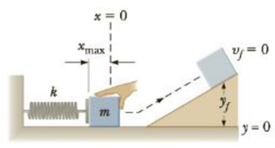Chapter 13, Problem 11P

Chapter
Section
Textbook Problem

A student pushes the 1.50-kg block in Figure P13.11 against a horizontal spring, compressing it by 0.125 m. When released, the block travels across a horizontal surface and up an incline. Neglecting friction, find the block’s maximum height if the spring constant is k = 575 N/m.Figure P13.11

To determine
The block’s maximum height.

Explanation

Given information:

The mass of the block is 1.50 Kg.

The horizontal spring compresses by 0.125 m.

The spring constant of the spring is 575Nm-1 .

In the absence of non-conservative forces, the law of conservation of energy, assuming y=0 to be the ground level of the potential, can be written as,

KEi+PEGi+PESi=KEf+PEGf+PESf (1)

• KEi is the kinetic energy in the initial state
• PEGi is the potential energy due to gravity at initial state
• PESi is the potential energy due to the spring at initial state
• KEf is the kinetic energy in the final state
• PEGf is the potential energy due to gravity at final state
• PESf is the potential energy due to the spring at final state

Consider the compressed position of the spring as the initial state and the position of maximum height of the block on the inclined plane as the final state

Still sussing out bartleby?

Check out a sample textbook solution.

See a sample solution

The Solution to Your Study Problems

Bartleby provides explanations to thousands of textbook problems written by our experts, many with advanced degrees!

Get Started